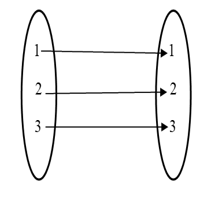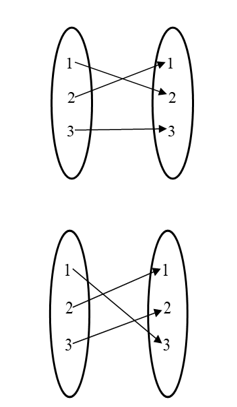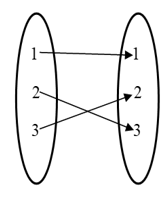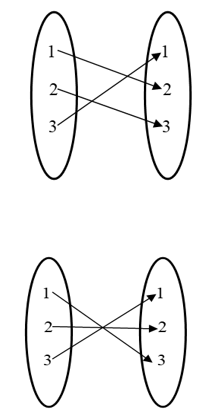#### Provide solution for RD Sharma maths class12 Chapter Functions exercise 2.1  question 16Given:

Here given that   is the set.

Here we have find all onto function from the given set.

Hint:

Since if f is onto then all element have a unique pre image.

Solution:

Taking the set

Total number of one-one function

As example since f is onto, all elements of  have unique pre-image

 Element Number of possible pairs 1 3 2 2 3 1So as the diagram, we get

 Elements Number of possible pairs 1 n 2 n-1 3 n-2 . . . . n-1 . n 2 1

So as the diagram, we get

#### infoexpert24